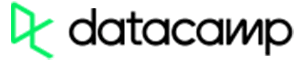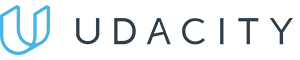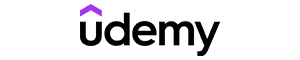Code has been added to clipboard!

# How to Create a Random Number Generator in C++

Published Oct 14, 2019
Updated Oct 14, 2019

The random number generator in C++ is a program that generates seemingly random numbers. You should learn how to create this feature if you need to make something in your program or website random. For instance, you might want your users to roll dice and receive rewards according to the number they get.

## Using the random number generator to choose prizes for users

You can easily imitate dice rolling by using a random number generator.

Let's say you are organizing a prize giveaway for your users. However, the prizes need to be selected randomly while also keeping users entertained. A random dice roll is a solid option.

The following example of the C++ code shows how the roll dice feature lets you randomly pick prizes for your users (according to the number rolled). For this aim, you should also use the switch statement to present different outcomes:

Example
``````#include <stdio.h>
#include <stdlib.h>
#include <ctime>

int main() {
srand((unsigned) time(0));
printf("Your dice has been rolled! You got: \n ");
int result = 1 + (rand() % 6);
printf("%d \n", result);
switch (result) {
case 1:
printf("Your prize is our original T-shirt!");
break;
case 2:
printf("Your prize is our original cap!");
break;
case 3:
printf("Your prize is our original necklace!");
break;
case 4:
printf("Your prize is our original keychain!");
break;
case 5:
printf("Your prize is our original cup set!");
break;
case 6:
printf("Your prize is a set of original keychains!");
break;
default:
printf("Error");
break;
}
}``````

The code example above represents a simple way of using the random number generator for real purposes. To learn more about the way such generators come to life, continue reading this tutorial.

## Where are random numbers used?

You can create a random number generator in C++ by using the `rand()` and `srand()` functions that come with the standard library of C++. Such a generator can have the starting number (the seed) and the maximum value.

Note: computers are all about correctness and predictability. Therefore, the random number selection is only imitated, not actually random. Thus, the selection is referred to as pseudo-random.

Learning how to generate random numbers in C++ is not difficult (but you should know the basics of C++). However, what is the practical use of a random number generator?

• The traditional use of random numbers is for adding them for game development. You can find random number generators in games involving dice, coins, or cards.
• It is possible to generate random quotes, jokes, or other content on websites by using the random number generators.
• Random numbers are often used in cryptography and cybersecurity.

## The main function for a random number generation

With the C++ `rand()` method, you can return a positive number within the range from 0.0 to `RAND_MAX`. If you want this function to work, you need to add the `<cstdlib>` header.

The following example shows how to create a program that generates one random number, which will be the same every time the program runs:

Example
``````#include <cstdlib>

#include <iostream>

using namespace std;

int main() {
int randomNumber = rand();
cout << randomNumber << endl;
}``````

The maximum value of the `rand()` is `RAND_MAX` (its minimum value is 32767). The value of `RAND_MAX` can differ according to the compiler. Check the value in your compiler with this code:

Example
``````#include <cstdlib>
#include <iostream>

using namespace std;

int main() {
cout << "RAND_MAX value is " << RAND_MAX << endl;
}``````

You can make C++ generate random numbers from a unique range by using the `%` modulus operator. This operator lets you set the maximum value that can be generated. The following example shows how you can return a random number between 1 and 35:

Example
``````#include <cstdlib>
#include <ctime>
#include <iostream>

using namespace std;

int main() {
srand((unsigned) time(0));
int randomNumber;
for (int index = 0; index < 10; index++) {
randomNumber = (rand() % 35) + 1;
cout << randomNumber << endl;
}
}``````

The following code example shows how you can imitate 20 rolls of a die:

Example
``````#include <stdio.h>
#include <stdlib.h>

int main() {
for (int i = 1; i <= 20; i++) {
printf("%d ", 1 + (rand() % 6));
if (i % 5 == 0) {
printf("\n");
}
}
}``````

You should notice that the outcome is always the same in the example above. This issue can be solved if you use the C++ `srand()` function:

Example
``````#include <stdio.h>
#include <stdlib.h>
#include <ctime>

int main() {
srand((unsigned) time(0));
for (int i = 1; i <= 20; i++) {
printf("%d ", 1 + (rand() % 6));
if (i % 5 == 0) {
printf("\n");
}
}
}``````

## Making the random numbers different after every execution

It is not enough to only use the `rand()` function to make the C++ generate random numbers.

If you do not use the `srand` method together with `rand`, you will get the same sequence every time code runs.

To avoid the repetitive sequence, you must set the seed as an argument to the `srand()` method. However, setting a fixed value for the `srand()` is also not a good option as the output remains the same.

A very useful tip to make C++ generate random numbers is to use the `time()` method. By seeding the generator with the same number, you are more likely to get the same random number each time.

Therefore, if you use the C++ `srand()` with the current time, the generated random number will always be different.

Example
``````#include <cstdlib>
#include <ctime>
#include <iostream>

using namespace std;

int main() {
srand((unsigned) time(0));
int randomNumber = rand();
cout << randomNumber << endl;
}``````

Tip: you need to call the C++ srand() function only once at the beginning of code (before calling rand()). Multiple calls can influence the generation of random numbers negatively.Pros
• Easy to use with a learn-by-doing approach
• Offers quality content
• Gamified in-browser coding experience
• The price matches the quality
• Suitable for learners ranging from beginner to advanced
Main Features
• Free certificates of completion
• Focused on data science skills
• Flexible learning timetablePros
• Simplistic design (no unnecessary information)
• High-quality courses (even the free ones)
• Variety of features
Main Features
• Nanodegree programs
• Suitable for enterprises
• Paid Certificates of completionPros
• Easy to navigate
• No technical issues
• Seems to care about its users
Main Features
• Huge variety of courses
• 30-day refund policy
• Free certificates of completion

## Generating numbers within different ranges

### Random numbers between 0 and 1

You can generate a C++ random number between 0 and 1 by combining `rand()`, `srand()`, and the modulus operator. The following example shows how you can generate random numbers from 0 to 1 (excluding 0 and 1):

Example
``````#include <time.h>
#include <iostream>

using namespace std;

int main() {
srand((unsigned) time(NULL));

for (int i = 0; i < 5; i++) {
cout << (float) rand() / RAND_MAX << endl;
}
}``````

The next code example shows how you can generate either 0 or 1:

Example
``````#include <time.h>
#include <iostream>

using namespace std;

int main() {
srand(time(NULL));
cout << rand() % 2;
}``````

### Random numbers between 1 and 10

You can generate a C++ random number between 1 and 10. The following example generates five random numbers within the range from 1 to 10 (including 1 and 10):

Example
``````#include <cstdlib>
#include <ctime>
#include <iostream>

using namespace std;

int main() {
srand((unsigned) time(0));
int randomNumber;
for (int index = 0; index < 5; index++) {
randomNumber = (rand() % 10) + 1;
cout << randomNumber << endl;
}
}``````

### Random numbers between 1 and 100

You can return a C++ random number between 1 and 100 as well by modifying the modulus operator:

Example
``````#include <cstdlib>
#include <ctime>
#include <iostream>

using namespace std;

int main() {
srand((unsigned) time(0));
int randomNumber;
for (int index = 0; index < 5; index++) {
randomNumber = (rand() % 100) + 1;
cout << randomNumber << endl;
}
}``````

Days
Hours
Minutes
Seconds